# Texas Go Math Grade 6 Lesson 4.2 Answer Key Dividing Decimals

Refer to our Texas Go Math Grade 6 Answer Key Pdf to score good marks in the exams. Test yourself by practicing the problems from Texas Go Math Grade 6 Lesson 4.2 Answer Key Dividing Decimals.

## Texas Go Math Grade 6 Lesson 4.2 Answer Key Dividing Decimals

Reflect

Question 1.
Multiple Representations When using models to divide decimals, when might you want to use grids divided into tenths instead of hundredths?
We can use grids divided into tenths if we divide decimals with one decimal place.

Question 2.
Check for Reasonableness How can you estimate to check that your quotient in A is reasonable?
We can estimate to check that our quotient is reasonable dividing the whole number which is closest to given decimal by already given whole number.

Divide

Question 3.Solution to this example is given belowGo Math Dividing Decimals Grade 6 Lesson 4.2 Question 4.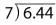Question 5.The divisor has one decimal, place, so multiply both the dividend and the divisor by 10 so that the divisor is a whole number

0.5 × 10 = 5
4.25 × 10 = 42.5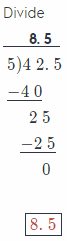Question 6.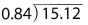The divisor has two decimal place, so mutliply both the dividend and the divisor by 100 so that the divisor is a whole number

0.84 × 100 = 84
15.12 × 100 = 1512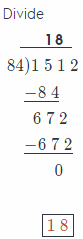Divide

Question 1.Here we will divide using long division as with whole numbers. will place a decimal point in the quotient directly above the decimal point in the dividend. So, we have the following: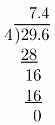So, the quotient is 7.4

Dividing Decimals Answer Key Go Math Lesson 4.2 Grade 6 Question 2.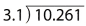Solution to this example is given below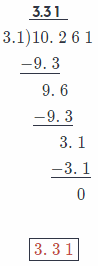Question 3.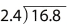The divisor has one decimal place, so multiply both the dividend and the divisor by 10 so that the divisor is a whole number

2.4 × 10 = 24
16.8 × 10 = 168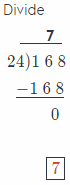Question 4.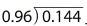The divisor has two decimal place, so multiply both the dividend and the divisor by 100 so that the divisor is a whole number

0.96 × 100 = 96
0.144 × 10 = 14.4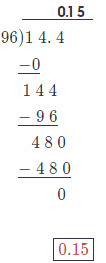Question 5.
38.5 ÷ 0.5 = _____________
The divisor has one decimal place, so multiply both the dividend and the divisor by 10 so that the divisor is a whole number

0.5 × 10 = 5
38.5 × 10 = 385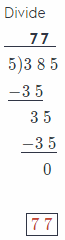Question 6.
23.85 ÷ 9 _____________
Solution to this example is given below5.6375 ÷ 0.71 _____________
The divisor has two decimal places, so multiply both the dividend and the divisor by 100 so that the divisor is a whole number

0.17 × 100 = 17
5.6372 × 100 = 563.72Question 8.
8.19 ÷ 4.2 _____________
The divisor has one decimal place, so multiply both the dividend and the divisor by 10 so that the divisor is a whole number

4.2 × 10 = 42
8.19 × 10 = 81.9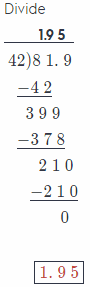Question 9.
66.5 ÷ 3.5 _____________
The divisor has one decimal place, so multiply both the dividend and the divisor by 10 so that the divisor is a whole number

3.5 × 10 = 35
66.5 × 10 = 665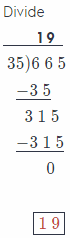Question 10.
0.234 ÷ 0.78 _____________
The divisor has two decimal place, so multiply both the dividend and the divisor by 100 so that the divisor is a whole number

0.78 × 100 = 78
0.234 × 100 = 23.4

Divide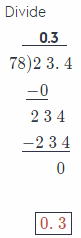Question 11.
78.74 ÷ 12.7 _____________
The divisor has one decimal place, so multiply both the dividend and the divisor by 10 so that the divisor is a whole number

12.7 × 10 = 127
78.74 × 10 = 787.4

Divide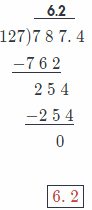Question 12.
36.34 ÷ 0.09 _____________
The divisor has two decimal place, so multiply both the dividend and the divisor by 100 so that the divisor is a whole number

0.09 × 100 = 9
36.45 × 100 = 3645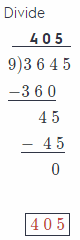Dividing Decimals for 6th Grade Go Math Question 13.
90 ÷ 0.36 _____________
The divisor has two decimal places, so multiply both the dividend and the divisor by 100 so that the divisor is a whole number

0.36 × 100 = 36
90 × 100 = 9000

Divide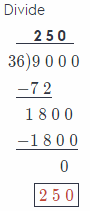Question 14.
18.88 ÷ 1.6 _____________
The divisor has one decimal place, so multiply both the dividend and the divisor by 10 so that the divisor is a whole number

1.6 × 10 = 16
18.88 × 10 = 188.8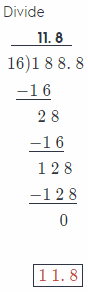Question 15.
Corrine has 9.6 pounds of trail mix to divide into 12 bags. How many pounds of trail mix will go in each bag?
The divisor has one decimal place, so multiply both the dividend and the divisor by 10 so that the divisor is a whole number

12 × 10 = 120
9.6 × 10 = 96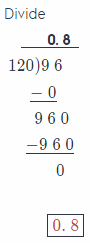Question 16.
Michael paid $1 1.48 for sliced cheese at the deli counter. The cheese cost$3.28 per pound. How much cheese did Michael buy?
The divisor has two decimal place, so multiply both the dividend and the divisor by 100 so that the divisor is a whole number

3.28 × 100 = 328
11.48 × 100 = 1148Question 17.
A four-person relay team completed a race in 72.4 seconds. On average, what was each runner’s time?
The divisor has one decimal place, so multiply both the dividend and the divisor by 10 so that the divisor is a whole number

4 × 10 = 40
72.4 × 10 = 724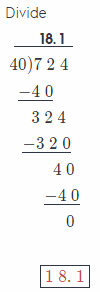Each person’s time 18.1 seconds

Question 18.
Elizabeth has a piece of ribbon that is 4.5 meters long. She wants to cut it into pieces that are 0.25 meter long. How many pieces of ribbon will she have?
The divisor has two decimal place, so multiply both the dividend and the divisor by 100 so that the divisor is a whole number

0.25 × 100 = 25
4.5 × 100 = 450

Divide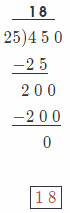Elizabeth will have 18 pieces of ribbon

Question 19.
Lisa paid $43.95 for 16.1 gallons of gasoline. What was the cost per gallon, rounded to the nearest hundredth? Answer: The divisor has one decimal place, so multiply both the dividend and the divisor by 10 so that the divisor is a whole number 16.1 × 10 = 161 43.95 × 10 =439.5Cost per gallon 2.73 dollars Question 20. One inch is equivalent to 2.54 centimeters. How many inches are there in 50.8 centimeters? Answer: The divisor has one decimal place, so multiply both the dividend and the divisor by 10 so that the divisor is a whole number 2.54 × 100 = 254 50.08 × 100 = 5008In 50.8 centimeters there is 20 inches Essential Question Check-In Question 21. How can you determine if you divided the numbers correctly? Answer: We can check it if we muLtipLy the resuLt by divisor. Multiplying those two numbers, we suppose to get the dividend. If we get the dividend, that means that we divided the number correctly. Multiplying the result by divisor. Use the table for 22 and 23.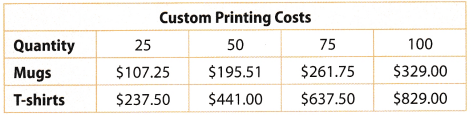Question 22. What is the price per mug for 25 coffee mugs? Answer: The solution to this example is given belowThe price per mug is 4.29 dollars Question 23. Find the price per T-shirt for 75 T-shirts. Answer: Solution to this example is given below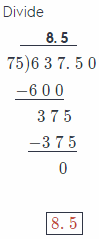The price per T-shirt is 8.5 dollars A movie rental website charges$5.00 per month for membership and $1.25 per movie. Texas Go Math Dividing Decimals Lesson 4.2 Grade 6 Question 24. How many movies did Andrew rent this month if this month’s bill was$16.25?
In order to calculate how many movies Andrew rented this month, we will first need to subtract 5.00 from the month’s bill, which is 16.25, and get:
16.25 – 5.00 = 11.25
Now, we will divide 11.25 by 1.25, but first, we need to change the divisor to a whole number by multiplying by a power of 100, and then multiply the dividend by the same power of 100 and get:So, Andrew rented 9 movies this month.

Question 25.
Marissa has $18.50 this month to spend on movie rentals. a. How many movies can she view this month? ___________________ Answer: In order to find how many movies Marissa can view, we will first subtract$ 5.00 for membership from $18.50 and get: 18.50 – 5.00 = 13.50 Now, we will divide 13.50 by 1.25, but first, we need to change the divisor to a whole number by multiplying by a power of 100, and then multiply the dividend by the same power of 100 and get: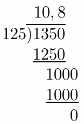We get that she can rent 10 movies. b. Critique Reasoning Marisa thinks she can afford 11 movies in one month. What mistake could she be making? Answer: In part (a) WP gets that She can rent 10.8 movies, but it is not a whole number. it is true that 11 is the closest whole number to 10.8. but Marissa does not have enough money for 11 movies, only for 10. Victoria went shopping for ingredients to make a stew. The table shows the weight and the cost of each of the ingredients that she bought.Question 26. What is the price for one pound of bell peppers? Answer: The divisor has two decimal place, so multiply both the dividend and the divisor by 100 so that the divisor is a whole number 2.50 × 100 = 250 1.25 × 100 = 125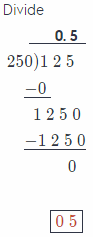Price for one pound of bell peppers is 0.5 dollars Question 27. Which ingredient costs the most per pound? Answer: Solution to this example is given belowBeef costs the most per pound. Question 28. What If? If carrots were$0.50 less per pound, how much would Victoria have paid for 8.5 pounds of carrots?
New cost of carrots
15.30 – 0.50 = 14.8Total cost of carrots for Vistoria is 125.80 dollars

Question 29.
Brenda is planning her birthday party. She wants to have 10.92 liters of punch, 6.5 gallons of ice cream, 3.9 pounds of fudge, and 25 guests at the birthday party.
a. Brenda and each guest drink the same amount of punch. How many liters of punch will each person drink? ______________________
Because Brenda has 10.92 liters of punch and 25 guests, we need to divide 10.92 by 26 in order to get how many liters of punch each person will drink, but first we need to change the divisor to a whole number by multiplying by a power of 100, and then multiply the dividend by the same power of 100.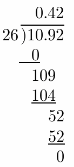So, we can notice that each person, including Brenda will drink 0.42 liters of punch.

b. Brenda and each guest eat the same amount of ice cream. How many gallons of ice cream will each person eat? ___________________
Brenda has 6.5 gallons of ice cream and 25 guests. so. we need to divide 6.5 by 26 in order to get how many gallons of ice cream each person will eat, but first we need to change the divisor to a whole number by multiplying by a power of 10, and then multiply the dividend by the same power of 10So, each person, including Brenda will eat 0.25 gallons of ice cream.

c. Brenda and each guest eat the same amount of fudge. How many pounds of fudge will each person eat? ____________________
Brenda has 3.9 pounds of fudge and 25 guests, so we need to divide 3.9 by 26 in order to get how many pounds of fudge each person will eat, but first We need to change the divisor to a whole number by multiplying by a power of 10. and then multiply the dividend by the same power of 10.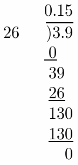So, each person including Brenda, will eat 0.15 pounds of fudge.

To make costumes for a play, Cassidy needs yellow and white fabric that she will cut into strips. The table shows how many yards of each fabric she needs, and how much she will pay for those yards.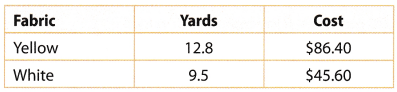Question 30.
Which costs more per yard, the yellow fabric or the white fabric?
We will first calculate how much costs the yellow fabric per yard dividing 86.40 by 12.8, hut first we need to change the divisor to a whole number by multiplying by a power of 10. and then multiply the dividend by the same power of 10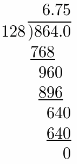So, the yellow fabric costs $6.75 per yard. Now, we will calculate how much costs the white fabric per yard dividing 45.60 by 9.5, but first we need to change the divisor to a whole number by multiplying by a power of 10, and then multiply the dividend by the same power of 10So, the white fabric costs$ 4.8 per yard.
Conclusion is that the yellow fabric costs more per yard.

Question 31.
Cassidy wants to cut the yellow fabric into strips that are 0.3 yards wide.
How many whole strips of yellow fabric can Cassidy make?
The divisor has one decimal place, so multiply both the dividend and the divisor by 10 so that the divisor is a whole number

0.3 × 10 = 3
12.8 × 10 = 128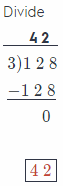Cassidy make 42 strips of yellow fabric.

H.O.T. Focus On Higher Order Thinking

Question 32.
Problem Solving Eight friends purchase various supplies for a camping trip and agree to share the total cost equally. They spend $85.43 on food,$32.75 on water, and $239.66 on other items. How much does each person owe? Answer: First, we will sum all their costs and get: 85.43 + 32.75 + 239.66 = 357.84 Now, we will divide total cost by 8 to calculate how much each person owes: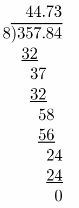So, each person owes$ 44.73

Question 33.
Analyze Relationships Constance ¡s saving money to buy a new bicycle that costs $195.75. She already has$40 saved and plans to save $8 each week. How many weeks will it take her to save enough money to purchase the bicycle? Answer: First we will subtract 10 form 195.75 and get: 195.75 – 40 = 155.75 Now, we will divide 155.75 by 8 in order to find how ninny weeks it will take Constance to save enough money to purchase the bicycle.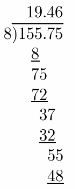So, it will take her about 20 weeks to save enough money for bicycle. Question 34. Represent Real-World Problems A grocery store sells twelve bottles of water for$13.80. A convenience store sells ten bottles of water for $1 1.80. Which store has the better buy? Explain. Answer: We will first calculate how much costs one bottle of water in the first store dividing 13.80 by 12 and get:So, in the first store. bottle of water costs S 1.15. Now we will calculate how much costs one bottle of water in the second store dividing 11.80 by 10 and get:In the second store, one bottle of water costs$ 1.18.
We can conclude that the better buy has the first store.

Scroll to Top